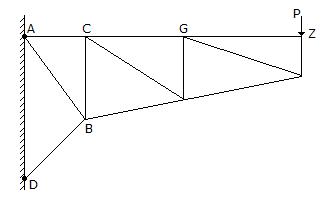# Civil Engineering - UPSC Civil Service Exam Questions

1.
For Froude number of a hydraulic jump is 5.5. The jump can be classified as a/an:
undularjump
oscillating jump
weak jump
Explanation:
No answer description is available. Let's discuss.

2.
A cantilever pin-jointed truss carries one load P at the point Z as shown in the given figure. The hinges in the vertical wall are at A and D. The truss has only three horizontal members AC, CG and GZ.
The nature of force in member ABis tensile
is zero
is compressive
cannot be predicted
Explanation:
No answer description is available. Let's discuss.

3.
A saturated stiff clay has unit weight 2 gm/cm3 and unconfined compressive strength 2 kg/cm2. The depth of tension crack that would develop in this clay is
2 m
5 m
10 m
20 m
Explanation:
No answer description is available. Let's discuss.

4.
Consider the following statements :
1. In a member subjected to uniaxial tensile force the maximum normal stress is the external load divided by the maximum cross-sectional area.
2. When the structural member is subjected to uniaxial loading, the shear stress is zero on a plane where the normal stress is maximum.
3. In a member subjected to uniaxial loading, the normal stress on the planes of maximum shear stress is less than the maximum.
Of these statements :
1 and 2 are correct
1 and 3 are correct
2 and 3 are correct
1, 2 and 3 are correct